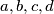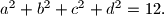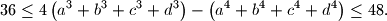### IMO Shortlist 2010 problem A2

Kvaliteta:
Avg: 0,0
Težina:
Avg: 6,0
Let the real numbers$a,b,c,d$ satisfy the relations$a+b+c+d=6$ and$a^2+b^2+c^2+d^2=12.$ Prove thatProposed by Nazar Serdyuk, Ukraine
Izvor: Međunarodna matematička olimpijada, shortlist 2010